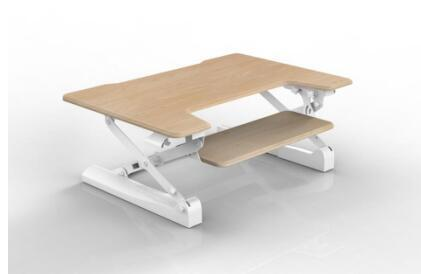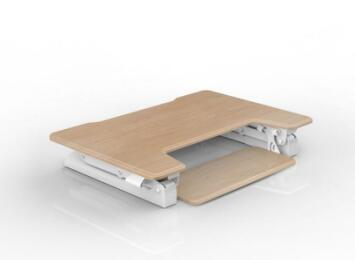|

# 随心升降新型站立办公捷昌J-AA01桌面升降器测评

前言：对于平均每天要坐8-12小时左右的上班一族来说，长期保持低头或固定前倾的坐姿办公，容易引发颈椎、腰椎疾病，体重上升以及许多慢性疾病。那么怎么减轻长时期坐着办公对自己的伤害？今天我就向大家推荐一款专业解决上述难题的产品——捷昌J-AA01桌面升降器，它是由捷昌研发从人体工学角度出发，专门为解决上述难题的新产品，倡导站立式办公的新型办公方式。是不是很期待这款J-AA01桌面升降器的性能到底如何，那就一起来体验一下吧！

1.产品外包装

*包装材质

*包装信息

捷昌J-AA01桌面升降器的外包装采用环保的瓦楞纸纸箱，包装外观设计得很简洁，印有清晰的产品商标、名称和各种产品信息以及产品的外观勾勒图，包装箱内泡沫板托、瓦楞纸板等保护措施非常到位。

2.产品外形

*2种配色

*圆角面板

*尺寸规格

按照目前来看，捷昌J-AA01桌面升降器至少拥有黑、白两色可选，黑色款采用黑色面板搭配黑色框架，一体感出色，酷劲感十足。白色款采用白色面板搭配灰色钢架，简洁亮眼，值得赞许。

面板采用圆角压边设计，表面处理得相当细腻，可以看出其“抠细节”的用心程度。当手肘支撑在上面打字操作时，感觉非常流畅。J-AA01带键盘托，推荐使用于主机键盘。桌板尺寸官方给出的是900*640mm，我测量的结果和捷昌官方给出的数据一致。升降台的升降范围官方的数值是150~500mm，升降行程为350mm，我实际的测量结果是150~505mm，升降行程为355mm，最高数值和官方略有差异，比官方给出的数值略高5mm，可见官方给出的数值略为保守一点，捷昌J-AA01实际的升降高度表现更出色。

办公类家具尺寸标准有700mm、720mm、740mm、760mm四个规格，捷昌J-AA01最大的高度是500mm，放在办公桌上以后就能达到1200mm以上，正好符合人体实际站立办公的高度需求，同时又能根据使用者身高自主调整到最佳使用高度。

3.性能与配置

*承重能力

*升降体验

*升降系统

桌面升降器主台面的最大承重在15kg，底盘是整个承重的受力点，要承载整个升降台、电脑乃至人体部分掌托重量，所以底盘的质量必须足够过关。捷昌J-AA01的底盘采用三面支撑式结构，受力均衡，能看得出制作工艺很精良，非常有高级感。底部做了防滑处理，且与桌面的贴合度很平整，不会产生晃动不稳的情况。

将底盘翻过来细看其构造，可以看到它的四个角都有圆形胶垫，胶垫的软硬度适中，可以起到很好的减震防滑作用。底盘所用的冷轧钢板都非常厚实，螺丝等部件看上去也非常牢固，这些都为底盘的承重能力提供了坚强的保障。底盘的表面采用了塑料外壳工艺，把内部的钢板及部件都包裹起来，整体外观更圆润整洁。

捷昌J-AA01的升降过程方便轻盈，握住手控把手和桌板用力一按，升降台随即升起，同样握住手控把手和桌板向下轻压，升降台随即下降。内置隐藏式气推杆、导轨，可实现13档固定调节，迎合150cm~190cm身高用户。顺畅的升降体验，得益于工程师的优化设计与协调的驱动构造。捷昌J-AA01的升降系统可以分为X形稳定支撑系统、气压助力系统、锁止系统三部分，三者分工明确，密切配合才有了捷昌J-AA01升降自如的表现。以上升桌板为例，当需要上升桌板的时候，用手按压锁止系统的扳手和桌板，锁止系统打开锁止状态，升降台在气压弹簧的弹力支撑下自动升起，升起的过程中，X形支撑系统的构造使得上升过程保持垂直的姿态，X形支撑的上部后方的支脚在钢珠滑轨内滑动，使整个升起过程非常的顺畅自如。当升降台上升到合适的高度后，松开锁止扳手，锁止系统锁定，桌板停止上升，停留在当前的高度。

捷昌J-AA01的气压助力系统是一根气弹簧，升降台向上升起的时候气弹簧能够提供助力，下降的时候则起到缓冲的作用。

锁止系统的扳手呈自然弯曲的弧形，符合人体工学结构，握感很舒适。锁止系统的钢索类似于自行车的闸线，看上去比自行车的闸线更粗，能承受更大的接拽力。升降台上下升降的时候，X形稳定系统的上部后方支脚在钢珠滑轨上作往复移动，一粒粒钢珠在灯光的照耀下闪闪发亮，钢珠滑轨的顺滑性使得升降过程更为顺畅。

捷昌J-AA01的X形稳定支撑系统的四个支脚，前面两个是固定的，后面两个会随着升降台的升降作前后往复运动，后上臂支脚附着在滑轨上起到顺滑的作用，后下臂支脚同样附着在滑轨上，也起顺滑的作用。后下臂支脚还有一个锁止的功能。锁止扳手控制的钢索末端在底盘上连接着一个弹簧，弹簧的头上固定着一块钢板，钢板与后下臂的横臂处于互相垂直的状态，钢板与后下臂的豁口正好对应，扳手没有扳动的时候，钢板正好插进豁口里面，X形稳定支撑系统的支脚就会被锁止，这个时候升降台不能升降。随着扳手的扳动，弹簧在钢索的牵引下会被压缩，，带动这块钢板收缩，脱离后下臂上面的豁口，这个时候X形稳定支撑系统的支脚就可以移动了，升降台也就能自由升降。总之，在测评过程中，你会发现捷昌J-AA01桌面升降器整个升降系统的设计是非常特殊巧妙的，值得打个高分。

总结：捷昌J-AA01是一款小而精巧，不占空间，适合放在桌面上体验新型站立式办公的桌面升降器。既有满满的“健康办公”情怀理念，同时精致的外观设计，出色的升降结构，轻便的操作体验等都是一种新突破。J-AA01，让我们在坐与站的办公之间，多了一种叫捷昌升降办公的选择。

`声明：本文由入驻焦点开放平台的作者撰写，除焦点官方账号外，观点仅代表作者本人，不代表焦点立场错误信息举报电话： 400-099-0099，邮箱：jubao@vip.sohu.com，或点此进行意见反馈，或点此进行举报投诉。`A B C D E F G H J K L M N P Q R S T W X Y Z
A - B - C - D - E
• A
• 鞍山
• 安庆
• 安阳
• 安顺
• 安康
• 澳门
• B
• 北京
• 保定
• 包头
• 巴彦淖尔
• 本溪
• 蚌埠
• 亳州
• 滨州
• 北海
• 百色
• 巴中
• 毕节
• 保山
• 宝鸡
• 白银
• 巴州
• C
• 承德
• 沧州
• 长治
• 赤峰
• 朝阳
• 长春
• 常州
• 滁州
• 池州
• 长沙
• 常德
• 郴州
• 潮州
• 崇左
• 重庆
• 成都
• 楚雄
• 昌都
• 慈溪
• 常熟
• D
• 大同
• 大连
• 丹东
• 大庆
• 东营
• 德州
• 东莞
• 德阳
• 达州
• 大理
• 德宏
• 定西
• 儋州
• 东平
• E
• 鄂尔多斯
• 鄂州
• 恩施
F - G - H - I - J
• F
• 抚顺
• 阜新
• 阜阳
• 福州
• 抚州
• 佛山
• 防城港
• G
• 赣州
• 广州
• 桂林
• 贵港
• 广元
• 广安
• 贵阳
• 固原
• H
• 邯郸
• 衡水
• 呼和浩特
• 呼伦贝尔
• 葫芦岛
• 哈尔滨
• 黑河
• 淮安
• 杭州
• 湖州
• 合肥
• 淮南
• 淮北
• 黄山
• 菏泽
• 鹤壁
• 黄石
• 黄冈
• 衡阳
• 怀化
• 惠州
• 河源
• 贺州
• 河池
• 海口
• 红河
• 汉中
• 海东
• I
• J
• 晋中
• 锦州
• 吉林
• 鸡西
• 佳木斯
• 嘉兴
• 金华
• 景德镇
• 九江
• 吉安
• 济南
• 济宁
• 焦作
• 荆门
• 荆州
• 江门
• 揭阳
• 金昌
• 酒泉
• 嘉峪关
K - L - M - N - P
• K
• 开封
• 昆明
• 昆山
• L
• 廊坊
• 临汾
• 辽阳
• 连云港
• 丽水
• 六安
• 龙岩
• 莱芜
• 临沂
• 聊城
• 洛阳
• 漯河
• 娄底
• 柳州
• 来宾
• 泸州
• 乐山
• 六盘水
• 丽江
• 临沧
• 拉萨
• 林芝
• 兰州
• 陇南
• M
• 牡丹江
• 马鞍山
• 茂名
• 梅州
• 绵阳
• 眉山
• N
• 南京
• 南通
• 宁波
• 南平
• 宁德
• 南昌
• 南阳
• 南宁
• 内江
• 南充
• P
• 盘锦
• 莆田
• 平顶山
• 濮阳
• 攀枝花
• 普洱
• 平凉
Q - R - S - T - W
• Q
• 秦皇岛
• 齐齐哈尔
• 衢州
• 泉州
• 青岛
• 清远
• 钦州
• 黔南
• 曲靖
• 庆阳
• R
• 日照
• 日喀则
• S
• 石家庄
• 沈阳
• 双鸭山
• 绥化
• 上海
• 苏州
• 宿迁
• 绍兴
• 宿州
• 三明
• 上饶
• 三门峡
• 商丘
• 十堰
• 随州
• 邵阳
• 韶关
• 深圳
• 汕头
• 汕尾
• 三亚
• 三沙
• 遂宁
• 山南
• 商洛
• 石嘴山
• T
• 天津
• 唐山
• 太原
• 通辽
• 铁岭
• 泰州
• 台州
• 铜陵
• 泰安
• 铜仁
• 铜川
• 天水
• 天门
• W
• 乌海
• 乌兰察布
• 无锡
• 温州
• 芜湖
• 潍坊
• 威海
• 武汉
• 梧州
• 渭南
• 武威
• 吴忠
• 乌鲁木齐
X - Y - Z
• X
• 邢台
• 徐州
• 宣城
• 厦门
• 新乡
• 许昌
• 信阳
• 襄阳
• 孝感
• 咸宁
• 湘潭
• 湘西
• 西双版纳
• 西安
• 咸阳
• 西宁
• 仙桃
• 西昌
• Y
• 运城
• 营口
• 盐城
• 扬州
• 鹰潭
• 宜春
• 烟台
• 宜昌
• 岳阳
• 益阳
• 永州
• 阳江
• 云浮
• 玉林
• 宜宾
• 雅安
• 玉溪
• 延安
• 榆林
• 银川
• Z
• 张家口
• 镇江
• 舟山
• 漳州
• 淄博
• 枣庄
• 郑州
• 周口
• 驻马店
• 株洲
• 张家界
• 珠海
• 湛江
• 肇庆
• 中山
• 自贡
• 资阳
• 遵义
• 昭通
• 张掖
• 中卫

1室1厅1厨1卫1阳台

1
2
3
4
5

0
1
2

1

1

0
1
2
3报名成功，资料已提交审核A B C D E F G H J K L M N P Q R S T W X Y Z
A - B - C - D - E
• A
• 鞍山
• 安庆
• 安阳
• 安顺
• 安康
• 澳门
• B
• 北京
• 保定
• 包头
• 巴彦淖尔
• 本溪
• 蚌埠
• 亳州
• 滨州
• 北海
• 百色
• 巴中
• 毕节
• 保山
• 宝鸡
• 白银
• 巴州
• C
• 承德
• 沧州
• 长治
• 赤峰
• 朝阳
• 长春
• 常州
• 滁州
• 池州
• 长沙
• 常德
• 郴州
• 潮州
• 崇左
• 重庆
• 成都
• 楚雄
• 昌都
• 慈溪
• 常熟
• D
• 大同
• 大连
• 丹东
• 大庆
• 东营
• 德州
• 东莞
• 德阳
• 达州
• 大理
• 德宏
• 定西
• 儋州
• 东平
• E
• 鄂尔多斯
• 鄂州
• 恩施
F - G - H - I - J
• F
• 抚顺
• 阜新
• 阜阳
• 福州
• 抚州
• 佛山
• 防城港
• G
• 赣州
• 广州
• 桂林
• 贵港
• 广元
• 广安
• 贵阳
• 固原
• H
• 邯郸
• 衡水
• 呼和浩特
• 呼伦贝尔
• 葫芦岛
• 哈尔滨
• 黑河
• 淮安
• 杭州
• 湖州
• 合肥
• 淮南
• 淮北
• 黄山
• 菏泽
• 鹤壁
• 黄石
• 黄冈
• 衡阳
• 怀化
• 惠州
• 河源
• 贺州
• 河池
• 海口
• 红河
• 汉中
• 海东
• I
• J
• 晋中
• 锦州
• 吉林
• 鸡西
• 佳木斯
• 嘉兴
• 金华
• 景德镇
• 九江
• 吉安
• 济南
• 济宁
• 焦作
• 荆门
• 荆州
• 江门
• 揭阳
• 金昌
• 酒泉
• 嘉峪关
K - L - M - N - P
• K
• 开封
• 昆明
• 昆山
• L
• 廊坊
• 临汾
• 辽阳
• 连云港
• 丽水
• 六安
• 龙岩
• 莱芜
• 临沂
• 聊城
• 洛阳
• 漯河
• 娄底
• 柳州
• 来宾
• 泸州
• 乐山
• 六盘水
• 丽江
• 临沧
• 拉萨
• 林芝
• 兰州
• 陇南
• M
• 牡丹江
• 马鞍山
• 茂名
• 梅州
• 绵阳
• 眉山
• N
• 南京
• 南通
• 宁波
• 南平
• 宁德
• 南昌
• 南阳
• 南宁
• 内江
• 南充
• P
• 盘锦
• 莆田
• 平顶山
• 濮阳
• 攀枝花
• 普洱
• 平凉
Q - R - S - T - W
• Q
• 秦皇岛
• 齐齐哈尔
• 衢州
• 泉州
• 青岛
• 清远
• 钦州
• 黔南
• 曲靖
• 庆阳
• R
• 日照
• 日喀则
• S
• 石家庄
• 沈阳
• 双鸭山
• 绥化
• 上海
• 苏州
• 宿迁
• 绍兴
• 宿州
• 三明
• 上饶
• 三门峡
• 商丘
• 十堰
• 随州
• 邵阳
• 韶关
• 深圳
• 汕头
• 汕尾
• 三亚
• 三沙
• 遂宁
• 山南
• 商洛
• 石嘴山
• T
• 天津
• 唐山
• 太原
• 通辽
• 铁岭
• 泰州
• 台州
• 铜陵
• 泰安
• 铜仁
• 铜川
• 天水
• 天门
• W
• 乌海
• 乌兰察布
• 无锡
• 温州
• 芜湖
• 潍坊
• 威海
• 武汉
• 梧州
• 渭南
• 武威
• 吴忠
• 乌鲁木齐
X - Y - Z
• X
• 邢台
• 徐州
• 宣城
• 厦门
• 新乡
• 许昌
• 信阳
• 襄阳
• 孝感
• 咸宁
• 湘潭
• 湘西
• 西双版纳
• 西安
• 咸阳
• 西宁
• 仙桃
• 西昌
• Y
• 运城
• 营口
• 盐城
• 扬州
• 鹰潭
• 宜春
• 烟台
• 宜昌
• 岳阳
• 益阳
• 永州
• 阳江
• 云浮
• 玉林
• 宜宾
• 雅安
• 玉溪
• 延安
• 榆林
• 银川
• Z
• 张家口
• 镇江
• 舟山
• 漳州
• 淄博
• 枣庄
• 郑州
• 周口
• 驻马店
• 株洲
• 张家界
• 珠海
• 湛江
• 肇庆
• 中山
• 自贡
• 资阳
• 遵义
• 昭通
• 张掖
• 中卫• 手机• 分享
• 设计
免费设计
• 计算器
装修计算器
• 入驻
合作入驻
• 联系
联系我们
• 置顶
返回顶部